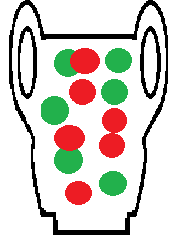# Pólya Distribution

## What is a Pólya Distribution?Urn models are simple ways to represent real life probabilities.The Pólya distribution (also called the Pólya-Eggenberger distribution), named after George Pólya, is a discrete probability distribution related to Pólya’s urn (also called the Pólya-Eggenberger urn scheme) . It describes the number of red balls drawn in the first n trials of Pólya’s urn. The number of black balls follows a negative Pólya-Eggenberger distribution .

The Pólya distribution has applications in fields as diverse as genetics, insurance, and modeling epidemics.

The multivariate Pólya distribution, sometimes called the Dirichlet-multinomial distribution or Dirichlet compound multinomial distribution, is an extension of the univariate beta binomial distribution.

## Process and PMF for the Pólya Distribution

The distribution models a simple process: draw a random ball from an urn containing r red balls and N r black balls. Record the color of the ball, then return the ball to the urn with c additional balls of the same color. Repeat the process for n draws. If X is the number of red balls removed in the first n trials, then the random variable X follows a Pólya distribution.

When the sample size is large enough, the Pólya distribution can be estimated with the binomial distribution.

## References

 Kaiser, H. & Stefansky, W. A Polya Distribution for Teaching. The Teacher’s Corner. Retrieved November 13, 2021 from: https://www.jstor.org/stable/2682866
 Marshall, A. (1990). Bivariate Distributions Generated from Pólya-Eggenberger Urn Models. Journal of Multivariate Analysis 35, 48-65.
Teerapabolarn, K. (2014). An Improved Binomial Distribution to Approximate the Pólya Distribution. International Journal of Pure and Applied Mathematics
Volume 93 No. 5, 629-632

CITE THIS AS:
Stephanie Glen. "Pólya Distribution" From StatisticsHowTo.com: Elementary Statistics for the rest of us! https://www.statisticshowto.com/polya-distribution/